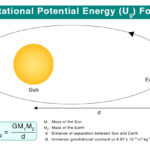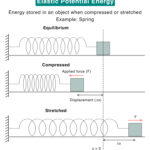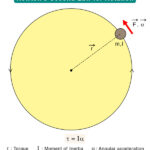Home / Physics / Magnetic Flux

# Magnetic Flux

## What is Magnetic Flux

Magnets and electromagnets produce a magnetic field represented by imaginary lines known as magnetic field lines or magnetic lines of force. Magnetic flux is the number of lines passing through a given area, whether in air or vacuum or inside a magnetic material. The magnetic flux is analogous to the electric flux.

## Magnetic Flux Equation

The magnetic flux is a scalar quantity and can be quantified by establishing an imaginary surface represented by an area vector in the vicinity of a magnetic field. The magnetic flux φ is given by the dot product of the magnetic field and the area vector.

$\phi = \vec{B}.\vec{A}$

If the angle between the magnetic field vector and the area vector is θ, then the magnetic flux is given by

$\phi = BA\cos \theta$

Symbol: Greek letter phi, φ

SI Unit: Weber or Wb

CGS Unit: Maxwell

Dimensions: [M1 L2 T-2 I-1]

When θ = 0o , φ = BA. Therefore, the flux is maximum when the area under consideration is perpendicular to the magnetic field.

Suppose the magnetic field and the area vector are in opposite directions, then the angle between the two is 180o and the flux will be negative.

Problem 1: A rectangular loop is 0.50 m long and 0.40 m wide. It is placed in a constant magnetic field of 0.3 T such that its area vector A makes an angle of 30° with B. Determine the magnetic flux through the surface.

Solution:

Given,

B = 0.3 T

θ = 30  ͦ

l = 0.5 m

w = 0.4 m

A = l x w = 0.5 x 0.4 = 0.2 m2

Therefore,

φ = BA cos θ

or, φ = 0.3 T x 0.2 m2 x cos 30  ͦ

or, φ = 0.052 Wb

Problem 2: A magnetic field of 1.5 T passes perpendicular to a disc of diameter 6 cm. Find the magnetic flux passing through the disc.

Solution:

Given,

B = 1.5 T

d = 6 cm

r = d/2 = 6/2 cm = 3 cm = 0.03 m

A = πr2 = π x (0.03 m)2 = 0.0028 m2

We have,

φ = BA

or, φ = 1.5 T x 0.0028 m2

or, φ = 0.0042 Wb

Magnetic Flux Density

In the above equation, when A = 1, φ = B. Therefore, the magnetic flux density is defined as the flux passing through a unit cross-sectional area.

Magnetic Field from Magnetic Flux

By rearranging the above equation, we get

$B = \frac{\phi}{A}$

Thus, the magnetic field is given by the flux per unit area perpendicular to the field.

SI Unit: Wb/m2

## Significance of Magnetic Flux

Magnetic flux is significantly essential in Faraday’s law of electromagnetic induction. Suppose a conducting wire is wound into a loop or a coil, and a magnet is brought closer. According to Faraday’s law, a current will be induced in the wire whose electromotive force or emf is given by,

$\epsilon = -N \frac{\Delta \phi}{\Delta t}$

where,

ε: Induced emf

N: Number of turns of the coil

Δφ/Δt: Change in magnetic flux over time

### Lenz’s Law

Suppose a loop of wire is placed in a changing magnetic field such that the magnetic flux passes through the wire. Then, Lenz’s law gives the direction of the induced current in the wire and the polarity of the induced emf. The induced current will generate an induced magnetic field, whose direction is given by the right-hand rule.

### Gauss’s Law for Magnetism

Gauss’s law for magnetism states that the net magnetic flux coming out of a closed surface is zero. This statement gives an understanding of magnetic field sources. For example, all field lines in a bar magnet from the north pole must enter the south pole. A closed surface enclosing a bar magnet will have no flux coming out. Therefore, Gauss’s law for magnetism proves that a magnetic monopole does not exist.

## FAQs

Q.1. What instrument is used to measure the magnetic flux?

Ans. A magnetometer is a device used to measure the magnetic field and the magnetic flux at a given point.

Article was last reviewed on Sunday, September 4, 2022

### Related articlesConservation of EnergyGravitational Potential EnergyElastic Potential EnergyNewton’s Second Law for Rotation

### One response to “Magnetic Flux”

1. SCAMORA SIMON says:

I really enjoyed it and its so fantastic that I would like to learn again next time